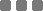## Tuesday, January 28, 2014

### Math: Finding the Value of sin18°∙sin54° without Calculator

A somewhat-funky Algebraic trick, which is interesting to me.

Let's find the value of sin 18°∙sin 54° without the calculator.

The things to remember are :
• The double angle formula for sine function, that is :
sin 2α = 2∙sin α∙cos α
• The co-function (0° ≤ α ≤ 90°), that is :
sin α = cos (90° - α) or cos α = sin (90° - α)

This brain teaser is actually an exercise in the section of sum of angles within the trigonometric function, especially for sine (double-angle) - senior high Math.

To "solve" sin 18°∙sin 54°, let's start from sin 72°.

Why 72°?

It's from 18° + 54° = 72°.

You'll see why I just added those two angles as the first step.

Look at this picture I made to watch where it will go :

Pretty much fun. The answer is 1/4 or 0.25.
This method only works for 18° and 54°. Both sine functions.
sin18°∙sin54° using co-function is equivalent with sin18°∙cos36° or cos72°∙sin54° or cos72°∙cos36°.

Anyway, I also made a JavaScript calculator for this particular post, for both sine functions, so you won't doubt the result above.
I round up the result to two decimal places. Try it out:
sin °∙ sin °

That is all. Thanks for visiting.

Related :Math: Finding the Value of sin18°∙sin54° without Calculator
https://monkeyraptor.johanpaul.net/2014/01/finding-value-of-sin18sin54-without.html